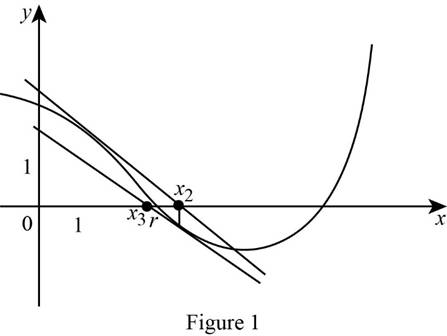# To draw: The graph of a tangent line at x 1 = 6 and estimate the numerical value of x 2 and x 3 from graph.### Single Variable Calculus: Concepts...

4th Edition
James Stewart
Publisher: Cengage Learning
ISBN: 9781337687805### Single Variable Calculus: Concepts...

4th Edition
James Stewart
Publisher: Cengage Learning
ISBN: 9781337687805

#### Solutions

Chapter 4.7, Problem 2E
To determine

## To draw: The graph of a tangent line at x1=6 and estimate the numerical value of x2 and x3 from graph.

Expert Solution

The numerical value of x2 and x3 is 3.5_ and 2.8_.

### Explanation of Solution

At first draw the tangent line at the point x1=1 and the tangent line intersect the x -axis. Let the intersecting point be x2.

Similarly, draw the tangent line at the point x2=3.5 and the tangent line intersect the x-axis. Let that intersecting point be x3.

Graph:Observation:

From Figure 1, it is observed that the value of x2 is equal to 3.5 and x3 is equal to 2.8 and also observed that the value of x3 is close to the value of r.

Thus, the value of x2 and x3 is 3.5_ and 2.8_ and the value of x3 is close to the value of r.

### Have a homework question?

Subscribe to bartleby learn! Ask subject matter experts 30 homework questions each month. Plus, you’ll have access to millions of step-by-step textbook answers!